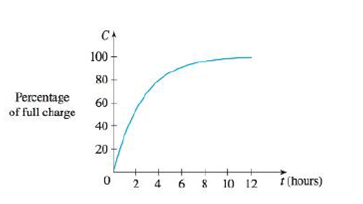Chapter 2.8, Problem 13E

Chapter
Section
Textbook Problem

A rechargeable battery is plugged into a charger. The graph shows C(t), the percentage of full capacity that the battery reaches as a function of time t elapsed (in hours).(a) What is the meaning of the derivative C'(t)?(b) Sketch the graph of C'(t). What does the graph tell you?(a)

To determine

To explain: The meaning of the derivative C(t).

Explanation

The meaning of derivative of C(t) is as follows:

This means that the ratio of change of percentage of full capacity in a very infinitesimal change of time

(b)

To determine

To sketch: The graph of C(t) and illustrate the graph.

Still sussing out bartleby?

Check out a sample textbook solution.

See a sample solution

The Solution to Your Study Problems

Bartleby provides explanations to thousands of textbook problems written by our experts, many with advanced degrees!

Get Started

Solve the equations in Exercises 126. (x21)2(x+2)3(x21)3(x+2)2=0

Finite Mathematics and Applied Calculus (MindTap Course List)

State the guidelines for modeling with equations.

Precalculus: Mathematics for Calculus (Standalone Book)

Name each polygon:

Elementary Technical Mathematics

True or False: 23xdx is an improper integral.

Study Guide for Stewart's Single Variable Calculus: Early Transcendentals, 8th

Explain what is meant by the concept that main effects and interactions are independent.

Research Methods for the Behavioral Sciences (MindTap Course List)